## Compressions and Stretches

### Learning Outcomes

• Graph Functions Using Compressions and Stretches.

Adding a constant to the inputs or outputs of a function changed the position of a graph with respect to the axes, but it did not affect the shape of a graph. We now explore the effects of multiplying the inputs or outputs by some quantity.

We can transform the inside (input values) of a function or we can transform the outside (output values) of a function. Each change has a specific effect that can be seen graphically.

## Vertical Stretches and Compressions

When we multiply a function by a positive constant, we get a function whose graph is stretched or compressed vertically in relation to the graph of the original function. If the constant is greater than 1, we get a vertical stretch; if the constant is between 0 and 1, we get a vertical compression. The graph below shows a function multiplied by constant factors 2 and 0.5 and the resulting vertical stretch and compression.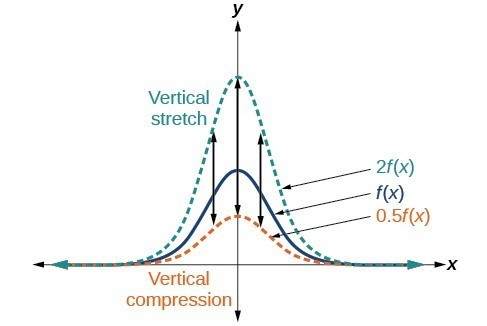Vertical stretch and compression

### A General Note: Vertical Stretches and Compressions

Given a function $f\left(x\right)$, a new function $g\left(x\right)=af\left(x\right)$, where $a$ is a constant, is a vertical stretch or vertical compression of the function $f\left(x\right)$.

• If $a>1$, then the graph will be stretched.
• If $0 < a < 1$, then the graph will be compressed.
• If $a<0$, then there will be combination of a vertical stretch or compression with a vertical reflection.

### How To: Given a function, graph its vertical stretch.

1. Identify the value of $a$.
2. Multiply all range values by $a$.
3. If $a>1$, the graph is stretched by a factor of $a$.
If ${ 0 }<{ a }<{ 1 }$, the graph is compressed by a factor of $a$.
If $a<0$, the graph is either stretched or compressed and also reflected about the $x$-axis.

### Example: Graphing a Vertical Stretch

A function $P\left(t\right)$ models the number of fruit flies in a population over time, and is graphed below.

A scientist is comparing this population to another population, $Q$, whose growth follows the same pattern, but is twice as large. Sketch a graph of this population.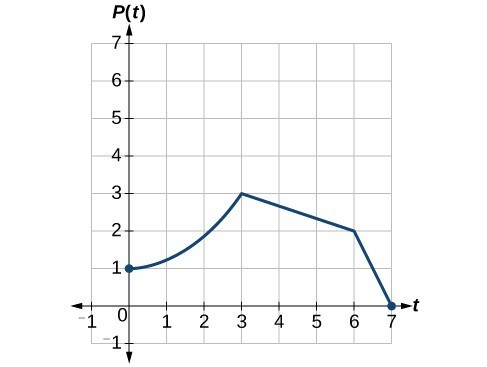### How To: Given a tabular function and assuming that the transformation is a vertical stretch or compression, create a table for a vertical compression.

1. Determine the value of $a$.
2. Multiply all of the output values by $a$.

### Example: Finding a Vertical Compression of a Tabular Function

A function $f$ is given in the table below. Create a table for the function $g\left(x\right)=\frac{1}{2}f\left(x\right)$.

 $x$ 2 4 6 8 $f\left(x\right)$ 1 3 7 11

### Try It

A function $f$ is given below. Create a table for the function $g\left(x\right)=\frac{3}{4}f\left(x\right)$.

 $x$ 2 4 6 8 $f\left(x\right)$ 12 16 20 0

### Example: Recognizing a Vertical Stretch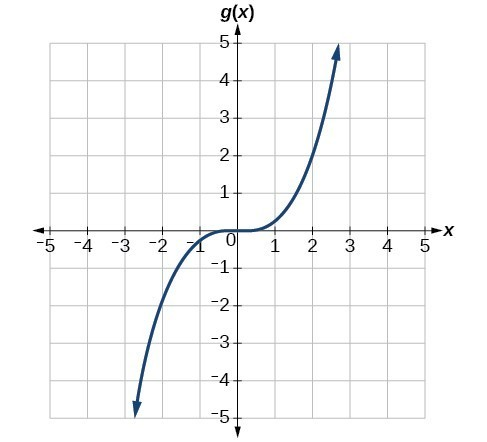The graph is a transformation of the toolkit function $f\left(x\right)={x}^{3}$. Relate this new function $g\left(x\right)$ to $f\left(x\right)$, and then find a formula for $g\left(x\right)$.

### Try It

Write the formula for the function that we get when we vertically stretch (or scale) the identity toolkit function by a factor of 3, and then shift it down by 2 units.
Check your work with an online graphing tool.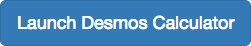## Horizontal Stretches and Compressions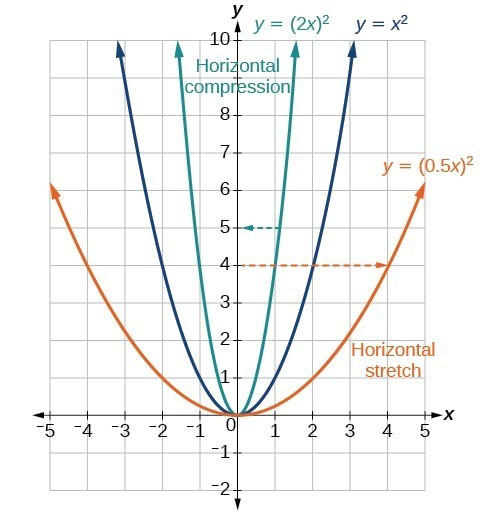Now we consider changes to the inside of a function. When we multiply a function’s input by a positive constant, we get a function whose graph is stretched or compressed horizontally in relation to the graph of the original function. If the constant is between 0 and 1, we get a horizontal stretch; if the constant is greater than 1, we get a horizontal compression of the function.

Given a function $y=f\left(x\right)$, the form $y=f\left(bx\right)$ results in a horizontal stretch or compression. Consider the function $y={x}^{2}$. The graph of $y={\left(0.5x\right)}^{2}$ is a horizontal stretch of the graph of the function $y={x}^{2}$ by a factor of 2. The graph of $y={\left(2x\right)}^{2}$ is a horizontal compression of the graph of the function $y={x}^{2}$ by a factor of 2.

### A General Note: Horizontal Stretches and Compressions

Given a function $f\left(x\right)$, a new function $g\left(x\right)=f\left(bx\right)$, where $b$ is a constant, is a horizontal stretch or horizontal compression of the function $f\left(x\right)$.

• If $b>1$, then the graph will be compressed by $\frac{1}{b}$.
• If $0<b<1$, then the graph will be stretched by $\frac{1}{b}$.
• If $b<0$, then there will be combination of a horizontal stretch or compression with a horizontal reflection.

### How To: Given a description of a function, sketch a horizontal compression or stretch.

1. Write a formula to represent the function.
2. Set $g\left(x\right)=f\left(bx\right)$ where $b>1$ for a compression or $0<b<1$
for a stretch.

### Example: Graphing a Horizontal Compression

Suppose a scientist is comparing a population of fruit flies to a population that progresses through its lifespan twice as fast as the original population. In other words, this new population, $R$, will progress in 1 hour the same amount as the original population does in 2 hours, and in 2 hours, it will progress as much as the original population does in 4 hours. Sketch a graph of this population.

### Example: Finding a Horizontal Stretch for a Tabular Function

A function $f\left(x\right)$ is given below. Create a table for the function $g\left(x\right)=f\left(\frac{1}{2}x\right)$.

 $x$ 2 4 6 8 $f\left(x\right)$ 1 3 7 11

### Example: Recognizing a Horizontal Compression on a Graph

Relate the function $g\left(x\right)$ to $f\left(x\right)$.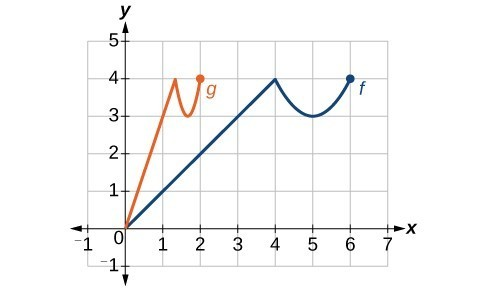### Try It

Write a formula for the toolkit square root function horizontally stretched by a factor of 3.
Use an online graphing tool to check your work.## Contribute!

Did you have an idea for improving this content? We’d love your input.